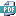#### 8th Grade & HS IM1

 ​(To: mateor@rps205.com ) ​Make-up Work Form ​​Home Access Center ​Textbook IM1 Homework IM1 ​Baño ​

VIDEOS​

9. ### ​Multiply powers​

10. Scientific notation​ (connect to the RPS205 network)
11. Adding & subtracting in scientific notation​ (connect to the RPS205 network)
12. Combining like terms with negative coefficients & distribution
13. Solving proportions​ (connect to the RPS205 network)
14. Number of solutions to equations​
15. Number of solutions to equations challenge​​
16. Intercepts from a graph (watch 2 videos: x-intercept & y-intercept​)
17. Pythagorean theorem proof​
8.EE.4
1. The scale of the universe - Interactive​
2. Scientific notation practice
3. Complete and show your work on a separate paper for ​​8.EE.3 WS Scientific notation.pdf​​.
4. XP Math Rewriting very large quantities using scientific notation​​​​
5. XP Math Rewriting very small quantities using scientific notation​
To Top